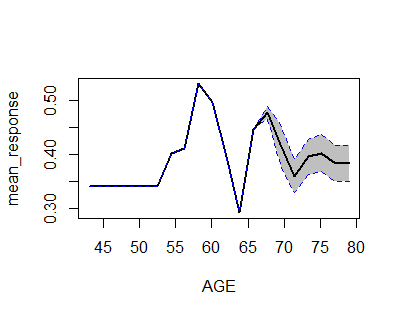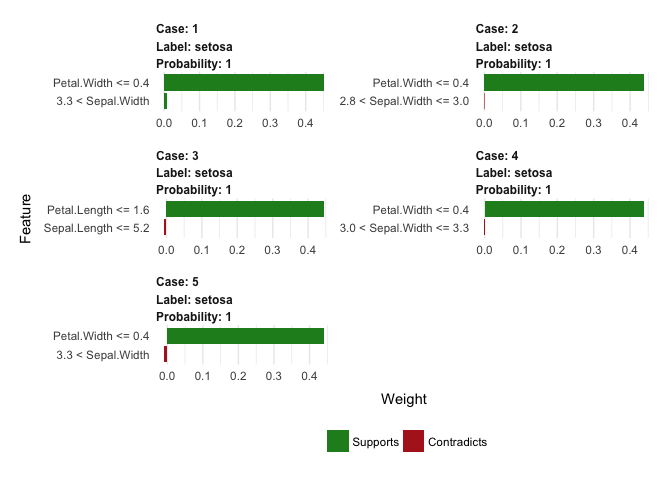2 views

I'm trying to explore the use of a GBM with h2o for a classification issue to replace a logistic regression (GLM). The non-linearity and interactions in my data make me think a GBM is more suitable.

I've run a baseline GBM (see below) and compared the AUC against the AUC of the logistic regression. THe GBM performs much better.

In a classic linear logistic regression, one would be able to see the direction and effect of each of the predictors (x) on the outcome variable (y).

Now, I would like to evaluate the variable importance of the estimate GBM in the same way.

How does one obtain the variable importance for each of the (two) classes?

I know that the variable importance is not the same as the estimated coefficient in logistic regression, but it would help me to understand which predictor impacts what class.

Others have asked similar questions, but the answers provided won't work for the H2O object.

Any help is much appreciated.

example.gbm <- h2o.gbm(

x = c("list of predictors"),

y = "binary response variable",

training_frame = data,

max_runtime_secs = 1800,

nfolds=5,

stopping_metric = "AUC")

by (33.1k points)

The advantages of GBM method also bring in difficulties to understand the model. This is valid for numeric variables, but when a GBM model utilises value ranges differently that some may have positive impacts whereas others have negative effects.

For GLM, if there is no specified interaction, then a numeric variable would be monotonic, hence you can have a positive or negative impact examed.

Partial Dependence Plot:

h2o provides h2o.partialplot that gives the partial effect for each variable

For example:

library(h2o)

h2o.init()

prostate.path <- system.file("extdata", "prostate.csv", package="h2o")

prostate.hex <- h2o.uploadFile(path = prostate.path, destination_frame = "prostate.hex")

prostate.hex[, "CAPSULE"] <- as.factor(prostate.hex[, "CAPSULE"] )

prostate.hex[, "RACE"] <- as.factor(prostate.hex[,"RACE"] )

prostate.gbm <- h2o.gbm(x = c("AGE","RACE"),

y = "CAPSULE",

training_frame = prostate.hex,

ntrees = 10,

max_depth = 5,

learn_rate = 0.1)

h2o.partialPlot(object = prostate.gbm, data = prostate.hex, cols = "AGE")

Output:### Using Individual Analyser:

There is a LIME package, that provides the capability to check variables contribution for each of the observations. This r package supports h2o already.